### Home > CALC > Chapter 5 > Lesson 5.3.3 > Problem5-136

5-136.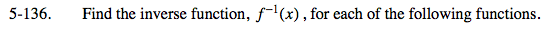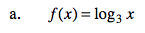Start by solving for x.
y = log3x
x = 3y
Now 'rename the x and y'.

f −1(x) = 3x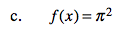Notice that y = π² is a horizontal line. What is the inverse of a horizontal line?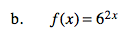y = 62x
2x = log6y

$x=\frac{\text{log}_6y}{2}$

f −1(x) = ____________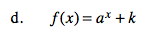Refer to hint in part (b).

Before you convert to a logarithm, subtract k from both sides.